## (TD) 1.11 Add a Free Parameter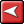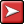## (TD) 1.11 Add a Free ParameterSo far we have always directly edited a parameter's values. This is inefficient and error prone if the circuit is large. It would be better to define one variable that held the value and make everything that needed that value reference this variable. Then if the value needs to be changed, you only have to edit one variable.

Free parameters act as variables that can be referenced by other parameters. They are called "free" because these parameters are not attached to any signal transitions in the Diagram window. Let's add a free parameter to hold the propagation times for the inverter.

 •In the Parameter window, press the Add Free Parameter button  to create a blank free parameter.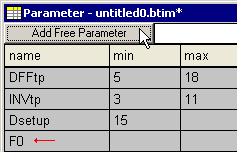•Double click on the free parameter to open the Parameter Properties dialog box and enter the following: Name is tpFreeInv, min time is 3ns, max time is 11ns, and comment is 74ALS04 inverter delay. (Leave the dialog open).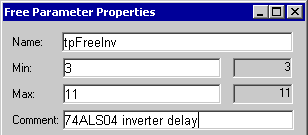•Make the dialog point to the INVtp delay, either by pressing the Previous button, or by double clicking on INVtp.•Type tpFreeInv into the min and max cells of INVtp and notice that the calculated values show the actual times. Any changes to the timing values of the free parameter will now affect this delay.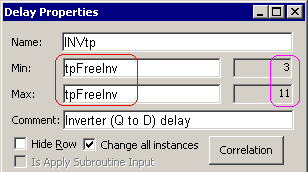•Notice that the row for tpFreeInv turned white to indicate that it is being referenced by another parameter.•Select the max value of INVtp in the parameter window and notice that the formula is displayed in the box above.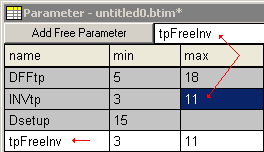•You can make the parameter window display formulas in the table part by choosing the Options > Parameter Window Preferences> Display min/max formula.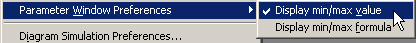Using Formulas in the Parameter time boxes:

Parameters can contain mathematical formulas as well as numeric time values. Common operations include multiplication(*), division(/), addition(+), and subtraction(-). For more information in the syntax for formulas see the Timing Diagram Editor Manual Section 2.5 Time Formulas for Clocks and Parameters.  For example, the inverter in this circuit could represent 3 cascaded inverters used to generate a minimum delay of 9ns. To represent this in your timing diagram:

 •Enter 3 * tbFreeInv into INVtp's min edit box. Then tab to a new box and see that the equation correctly calculated 9ns.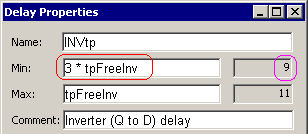•Free parameter names can also be used with an attributed parameter name such as tpFreeInv.min and tpFreeInv.max. This gives you the flexibility to specify formulas any way you need. If no attribute is added then a min or max is assumed depending on whether the formula is in the min or max column.

Create Libraries of Free Parameters:

Free parameters can be saved to special library files which can later be merged into other projects. You can also reference free parameters without including them into your project file by placing libraries in your library search path (Libraries > Library Preferences menu option). For more information on free parameters and libraries read the on-line help Chapter 10: Libraries or perform the Parameter Libraries Tutorial.